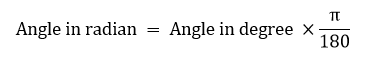Degrees to radians: In geometry, both degree and radian represent the measure of an angle. One complete anticlockwise revolution can be represented by 2π (in radians) or 360° (in degrees). Therefore, degree and radian can be equated as:

2π = 360°

And

π = 180°

Hence, from the above equation, we can say, 180 degrees is equal to π radian.

Usually, in general geometry, we consider the measure of the angle in degrees (°). Radian is commonly considered while measuring the angles of trigonometric functions or periodic functions. Radians is always represented in terms of pi, where the value of pi is equal to 22/7 or 3.14.

A degree has its sub-parts also, stated as minutes and seconds. This conversion is a major part of Trigonometry applications.

In this article, we are going to learn the conversion from degrees to radians, radians to degrees with its conversion procedure and many solved examples in detail.

## How to Convert Degrees to Radians?

The value of 180° is equal to π radians. To convert any given angle from the measure of degrees to radians, the value has to be multiplied by π/180.Where the value of π = 22/7 or 3.14

The below steps show the conversion of angle in degree measure to radians.

Step 1: Write the numerical value of the measure of an angle given in degrees

Step 2: Now, multiply the numeral value written in step 1 by π/180

Step 3: Simplify the expression by cancelling the common factors of the numerical

Step 4: The result obtained after the simplification will be the angle measure in radians

Let’s have a look at the examples of conversions of different angle measures.

Example 1: Convert 90 degrees to radians.

Solution: Given, 90 degrees is the angle

Angle in radian = Angle in degree x (π/180)

= 90 x (π/180)

= π/2

Hence, 90 degrees is equal to π/2 in radian.

As we have already discussed, how to convert degrees to radians for any specific angle. Now, let us see how we can convert radians to degrees for any specific angle. The formula to convert radians to degrees is given by:

Example 2: Convert π/6 into degrees.

Solution: Using the formula,

π/6 × (180/π) = 180/6 = 30 degrees

As we know already, one complete revolution, counterclockwise, in an XY plane, will be equal to 2π (in radians) or 360° (in degrees). Therefore, both degree and radian can form an equation, such that:

2π = 360°

Or

π = 180°

To convert degrees to radian, we can use the same formula as given in the above section.

Let us see some examples:

Example 3: Convert 15 degrees to radians.

Solution: Using the formula,

15 x π/180 = π/12

Example 4: Convert 330 degrees to radians.

Solution: Using the formula,

330 x π/180 = 11π/6

The method to convert a negative degree into radian is the same as we have done for positive degrees. Multiply the given value of the angle in degrees by π/180.

Suppose, -180 degrees has to be converted into radian, then,

Angle in radian = – π

To convert the angle value from degrees to radians, the calculator will help in quick results.

Let us create the table to convert some of the angles in degree form to radian form.

Angle in Degrees Angle in Radians
0

## Conversion Of Some Common Angles

Go through the table given below to understand the conversion of angles in comparison with the amount of turn.

## Solved Examples

Question 1: Convert 200 degrees into radians.

Solution: By the formula, we know;

Angle in radians = Angle in degree × π/180

Thus,

200 degrees in radians = 200 × π/180 = 10π/9 = 3.491 Rad

Question 2: Convert 450 degrees into radians.

Solution: By the formula, we know;

Angle in radians = Angle in degree × π/180

Thus,

### Practice Questions

1. Transform 700 degrees to radians.
2. How many radians is equal to 100 degrees?
3. Convert 550 degrees to radians.

### How do we convert degrees to radians?

To convert the value of the angle in degree, to its equivalent radians, we need to multiply the given value with π/180. For example, the value of 180 degrees is π in radians.

### What is the formula to convert radian into degrees?

To convert the value of angle from radian to degrees, we need to multiply the given value with 180/π. For example, the value of 2π:
2π x 180/π = 360 degrees

### How to convert 30 degrees to radians?

Multiply 30 degrees to π/180:
30 x (π/180) = π/6
Hence, 30 degrees is equal to π/6 in radians

### How pi radians is equal to 180 degrees?

One complete revolution, counterclockwise, in an XY plane, will be equal to 2π (in radians) or 360° (in degrees). Hence, we can write:
2π = 360°
Or
π = 180°
Therefore, pi is equal to 180 degrees

### What is the value of 1 degree?

One degree is equal to 0.0174533 radians.

### What is the value of 1 radian?

One radian is equal to 57.2958 degrees.

### Convert 3 radians to degrees.

3 radian × 180/π = 171.887Deg